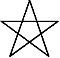There are eight months in the Calendar of Mu, sometimes referred to as the Nyarzirian Calendar. Each month consists of forty-five days, and represents a system of the eight hexagrams moving through the five elements. The Ninzuwu Priest/Priestess invokes the mudra/mantra formulae associated with each hexagram that rules a particular day after invoking the Armor of Amaterasu Ohkami. The chart below illustrates the daily Vasuh letters invoked and the ruling “deity” for the said hexagram:
1. August 1st 2016 = Kotoamatsukami = 1st Hexagram = Zhee-Shki
2. August 2nd 2016 = Suzha = 6th Hexagram = Hmu-Shki
3. August 3rd 2016 = Kamuy-Zi = 10th Hexagram = Lewhu
4. August 4th 2016 = Kayra-Jin = 12th Hexagram = Zhee-Shki-Zhee
5. August 5th 2016 = Ichikishimahime-no-Mikoto = 13th Hexagram = Shki-Aum-Zhee-Bnhu
6. August 6th 2016 = Ishitijin = 25th Hexagram = Zhee-Bnhu
7. August 7th 2016 = Naziqjin = 33rd Hexagram = Shki-Phe
8. August 8th 2016 = Inari-Ohkami = 44th Hexagram = Hmu-Hmu
9. August 9th 2016 =Entering Earth Element
10. August 10th 2016 = Kotoamatsukami = 1st Hexagram = Zhee-Shki
11. August 11th 2016 = Suzha = 6th Hexagram = Hmu-Shki
12. August 12th 2016 = Kamuy-Zi = 10th Hexagram = Lewhu
13. August 13th 2016 = Kayra-Jin = 12th Hexagram = Zhee-Shki-Zhee
14. August 14th 2016 = Ichikishimahime-no-Mikoto = 13th Hexagram = Shki-Aum-Zhee-Bnhu
15. August 15th 2016 = Ishitijin = 25th Hexagram = Zhee-Bnhu
16. August 16th 2016 = Naziqjin = 33rd Hexagram = Shki-Phe
17. August 17th 2016 = Inari-Ohkami = 44th Hexagram = Hmu-Hmu
18. August 18th 2016 =Entering Water Element
19. August 19th 2016 = Kotoamatsukami = 1st Hexagram = Zhee-Shki
20. August 20th 2016 = Suzha = 6th Hexagram = Hmu-Shki
21. August 21st  2016 = Kamuy-Zi = 10th Hexagram = Lewhu
22. August 22nd  2016 = Kayra-Jin = 12th Hexagram = Zhee-Shki-Zhee
23. August 23rd  2016 = Ichikishimahime-no-Mikoto = 13th Hexagram = Shki-Aum-Zhee-Bnhu
24. August 24th 2016 = Ishitijin = 25th Hexagram = Zhee-Bnhu
25. August 25th  2016 = Naziqjin = 33rd Hexagram = Shki-Phe
26. August 26th  2016 = Inari-Ohkami = 44th Hexagram = Hmu-Hmu
27. August 27th 2016 =Entering Fire Element
28. August 28th 2016 = Kotoamatsukami = 1st Hexagram = Zhee-Shki
29. August 29th 2016 = Suzha = 6th Hexagram = Hmu-Shki
30. August 30th 2016 = Kamuy-Zi = 10th Hexagram = Lewhu
31. August 31st  2016 = Kayra-Jin = 12th Hexagram = Zhee-Shki-Zhee
32. September 1st 2016 = Ichikishimahime-no-Mikoto = 13th Hexagram = Shki-Aum-Zhee-Bnhu
33. September 2nd 2016 = Ishitijin = 25th Hexagram = Zhee-Bnhu
34. September 3rd  2016 = Naziqjin = 33rd Hexagram = Shki-Phe
35. September 4th 2016 = Inari-Ohkami = 44th Hexagram = Hmu-Hmu
36. September 5th 2016 =Entering Air Element
37. September 6th 2016 = Kotoamatsukami = 1st Hexagram = Zhee-Shki
38. September 7th 2016 = Suzha = 6th Hexagram = Hmu-Shki
39. September 8th 2016 = Kamuy-Zi = 10th Hexagram = Lewhu
40. September 9th 2016 = Kayra-Jin = 12th Hexagram = Zhee-Shki-Zhee
41. September 10th 2016 = Ichikishimahime-no-Mikoto = 13th Hexagram = Shki-Aum-Zhee-Bnhu
42. September 11th 2016 = Ishitijin = 25th Hexagram = Zhee-Bnhu
43. September 12th 2016 = Naziqjin = 33rd Hexagram = Shki-Phe
44. September 13th 2016 = Inari-Ohkami = 44th Hexagram = Hmu-Hmu
45. September 14th 2016 =Entering Void Element

## 1 thought on “The Sign of Johuta 18,004 Begins: August 1st 2016”

1.thecelestialmaiden says:

Wafubeh and blessings as we enter the Sign of Johuta!